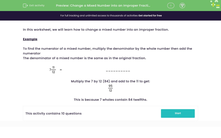# Change a Mixed Number into an Improper Fraction

In this worksheet, students will change a mixed number into an improper fraction.Key stage:  KS 3

Curriculum topic:   Number

Curriculum subtopic:   Use Four Operations for All Numbers

Difficulty level:#### Worksheet Overview

In this activity, we will learn how to change a mixed number into an improper fraction.

Example

To find the numerator of a mixed number, multiply the denominator by the whole number and then add on the numerator.

The denominator of a mixed number is the same as in the original fraction.

7
 11 12

In the example above, we need to multiply the whole number 7 by the denominator of 12. This is to find out how many twelfths there are in 7 wholes.

7 x 12 = 84  So, 7 wholes contain 84 twelfths.

Then, we need to add on the 11 twelfths that is the numerator: 84 + 11 = 95

The denominator remains unchanged as 12.

So, our improper fraction is:

 95 12Here's another one for you to check out.

What is 3 4/9 as an improper fraction?

First of all, we want to find out how many ninths there are in 3 whole numbers, so we multiply 3 by 9

3 x 9 = 27 so we know that there are 27 ninths in 3 whole numbers.

But we have 4 more ninths to include (the numerator) so we do 27 + 4 = 31

So we have 31 ninths in total which we write as 31/9

Let's have a go at some questions now.

### What is EdPlace?

We're your National Curriculum aligned online education content provider helping each child succeed in English, maths and science from year 1 to GCSE. With an EdPlace account you’ll be able to track and measure progress, helping each child achieve their best. We build confidence and attainment by personalising each child’s learning at a level that suits them.

Get started